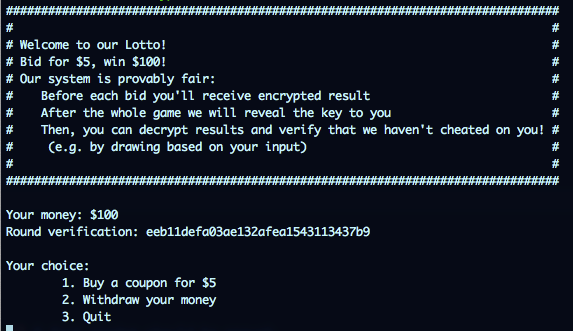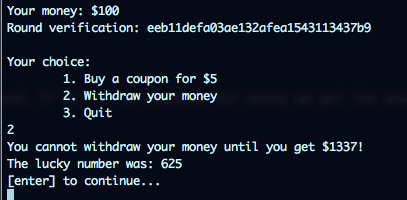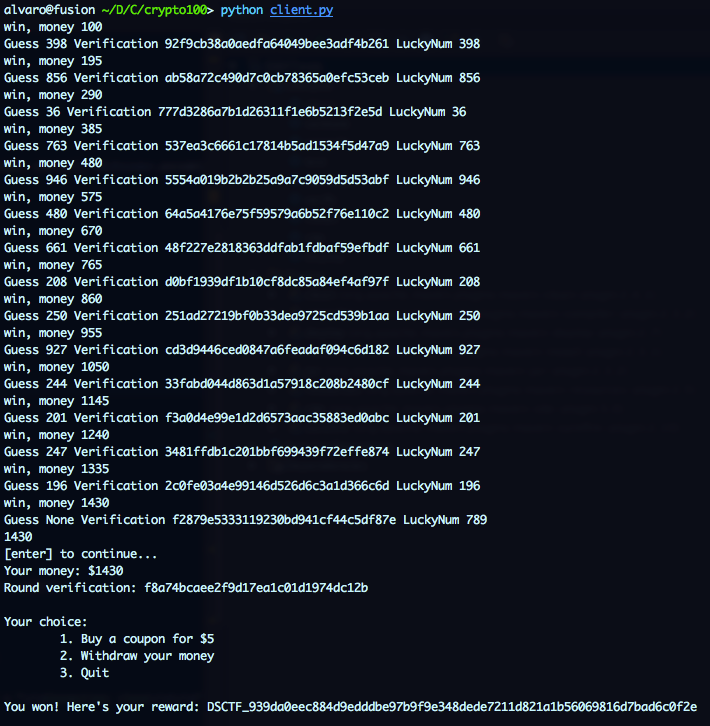In this task we have to win a lottery game:Basically each coupon costs $5 and we have$100 to spend. If we try to withdraw our money we get the amount of money we need to get our flag:To show they are playing fairly, the give you a verification id that its the value you have to guess concatenated with a random salt to reach the AES 16 bytes block that is used to encrypt the string. So we get:

AES(<number to guess>#random_salt, ECB_MODE)

We are also given the source code where we can verify this:

from Crypto.Cipher import AES
from Crypto import Random
from datetime import datetime
import random
import os
import time
import sys

# config
start_money = 100
cost = 5     # coupon price
reward = 100 # reward for winning
maxNumber = 1000 # we're drawing from 1 to maxNumber
screenWidth = 79

intro = [
'',
'Welcome to our Lotto!',
'Bid for $%d, win$%d!' % (cost, reward),
'Our system is provably fair:',
'   Before each bid you\'ll receive encrypted result',
'   After the whole game we will reveal the key to you',
'   Then, you can decrypt results and verify that we haven\'t cheated on you!',
'    (e.g. by drawing based on your input)',
''
]

# expand to AES block with random numeric salt
def randomExtend(block):
limit = 10**(16-len(block))
# salt
rnd = random.randrange(0, limit)
# mix it even more
rnd = (rnd ** random.randrange(10, 100)) % limit
# append it to the block
return block + ('%0'+str(16-len(block))+'x')%rnd

def play():
# print intro
print '#' * screenWidth
for line in intro:
print  ('# %-' + str(screenWidth-4) + 's #') % line
print '#' * screenWidth
print ''

# prepare everything
money = start_money

key = Random.new().read(16) # slow, but secure
aes = AES.new(key, AES.MODE_ECB)

# main loop
quit = False
while money > 0:
luckyNumber = random.randrange(maxNumber + 1) # fast random should be enough
salted = str(luckyNumber) + '#'
salted = randomExtend(salted)

print 'Your money: $%d' % money print 'Round verification: %s' % aes.encrypt(salted).encode('hex') print '' print 'Your choice:' print '\t1. Buy a coupon for$%d' % cost
print '\t3. Quit'

while True:
input = raw_input().strip()
if input == '1':
# play!
money -= cost
sys.stdout.write('Your guess (0-%d): ' % maxNumber)
guess = int(raw_input().strip())
if guess == luckyNumber:
print 'You won $%d!' % reward money += reward else: print 'You lost!' break elif input == '2': # withdraw if money > 1337: print 'You won! Here\'s your reward:', flag else: print 'You cannot withdraw your money until you get$1337!'
break
elif input == '3':
quit = True
break
else:
print 'Unknown command!'

print 'The lucky number was: %d' % luckyNumber
if quit:
break
print '[enter] to continue...'
raw_input()

print 'Verification key:', key.encode('hex')
if money <= 0:
print 'You\'ve lost all your money! get out!'

if __name__ == '__main__':
play()


The problem is that we cannot break AES, so we have to outsmart the system in a different way. There are two factors here that can help us with that:

First, the random salt appended to the value to guess is supposed to prevent us from creating a dictionary from Encrypted values to decrypted ones. Since the same value to guess will have many encrypted representations because of the salt appended. So here is the first mistake of the developers. The salt appended to the value to guess is not that random and turns out to be 000000000 many times because of the way the salt is calculated:

# expand to AES block with random numeric salt
def randomExtend(block):
limit = 10**(16-len(block))
# salt
rnd = random.randrange(0, limit)
# mix it even more
rnd = (rnd ** random.randrange(10, 100)) % limit
# append it to the block
return block + ('%0'+str(16-len(block))+'x')%rnd


Gynvael explained after the CTF was over than the reason for this was that:

Any number with a 0 as the last digit (i.e. 10% of numbers) rised to a high power will have all 000000000 at end and it gets truncated to % limit characters basically

The second factor is to find the way to play for free and I already showed you how to do it in the second screenshot. For each round we are presented with a verification value and after we choose an option, the value chosen is presented so we can verify that they were not cheating (although they dont give you the key so they could be cheating :D). Anyway, the second option, the one that lets us withdraw money works in the same way and so we can use it to know the number associated to an encrypted value.

So with that, we should be able to play and if we dont know the value associated to the encrypted value presented, we can ask for the withdraw process to get the lucky number associated to the crypto value and add them to a Encrypted-number map. If the encrypted value presented is in our map, then we can bet and win $100. Repeating the process can get us more than$1337 in less than 20 minutes

import socket

buffer = ""
while text not in buffer:
buffer = buffer + s.recv(1)
return buffer

host = "23.253.207.179"
port = 10001
s = socket.socket(socket.AF_INET, socket.SOCK_STREAM)
s.connect((host, port))

def play_round(mydict):
store = False
if encrypted in mydict:
guess = mydict[encrypted]
else:
guess = None
store = True
if guess is not None:
# Bet
s.send("1\n")
try:
guess = int(guess)
except:
guess = 1
s.send("{0}\n".format(guess))
else:
# Pass
s.send("2\n")
response = read_until(s,"The lucky number was: ")
if store:
mydict[encrypted] = num
if "won" in response:
print "win, money %d" % money
print "Guess %s Verification %s LuckyNum %s" % (str(guess), encrypted, num,)
if money > 1337:
print money
print num
s.send("\n")
s.send("2\n")
exit()
if "lost" in response:
print "WTF"
exit()